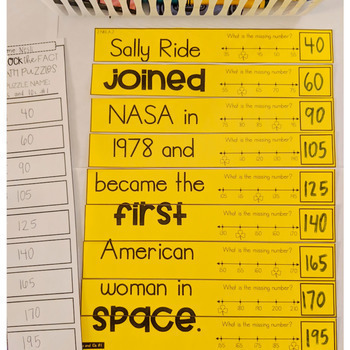# 2nd Grade Math Games | 2nd Grade Math Centers | 2nd Grade Math Puzzles | MarchResource Type
File Type
PDF (46 MB|90 pages)
Standards
\$4.00
Also included in:
1. These 2nd grade math centers and math interactive notebook cut and paste activities focus on the 2nd grade common core math standards and are perfect for guided math rotations or math workshop!________________________________Save money, buy the 2nd Grade bundle.3rd Grade bundle.©Unravel the Fact Mat
\$42.00
\$30.00
Save \$12.00
• Product Description
• Standards

©Unravel the Fact Math Puzzles are an original idea by The Teacher Wears Prada.

How do these low-prep math puzzles work?

The MARCH EDITION of these cross-curricular Unravel the Fact Math Puzzles reveal facts themed around holidays in March. Students will answer the 9 math problems, put the puzzle strips in order from LEAST to GREATEST. If they have answered the problems correctly and have placed the numbers in order correctly, an interesting fact will be revealed. They will have "unraveled a fact".

Content Integration + Self-Correcting Math Puzzles = Happy Teacher and Engaged Students

What themes/facts are featured this month?

Women's History Month/Amazing Women in History, History and Fun Facts about St. Patrick's Day, Spring Equinox, Facts about Pigs for National Pig Day, Facts about Pandas for National Panda Day, Facts about Butterflies for National Let's Learn about Butterflies Day

This resource includes 53 different math puzzles {two different formats} that cover a variety of 2nd Grade Common Core Math Skills. A recording sheet for accountability and a fact booklet for students to record their facts {optional} are also included.

Two different formats included:

Option #1: {MATH CENTERS} Print on colored paper, laminate, students write and wipe the answers with a thin dry erase marker. These laminated puzzles can be placed in math centers.

OR

Option #2: COMING SOON {PRINT AND GO RESOURCE} Pieces are already mixed up/jumbled up for students to solve, cut and put together to glue into math notebook or on a piece of construction paper. Students can complete these independently for morning work, math warm-ups, independent math work during math rotations, etc.

What skills are included?

{The Common Core standard is printed in the upper left corner of each puzzle}

• Telling Time (Half Hour)
• Telling Time (Quarter Hour)
• Telling Time (Five Minutes)
• Counting Money (pennies, nickels, dimes, quarters)
• Identifying Fractions
• Counting by 5s and 10s on a Number Line
• Counting by 100s
• Before and After (3 Digit Numbers)
• 3 Addends (two digits and single digit)
• Doubles Facts (Subtraction)
• Single Digit Subtraction Facts
• Mentally Subtract 10
• Mentally Subtract 100
• Even and Odd
• Plus 1, Minus 1, Plus 10, Minus 10 with 3 Digit Number
• 2 Digit Addition (Without Regrouping)
• 2 Digit Subtraction (Without Regrouping)
• 2 Digit Addition (With Regrouping)
• 2 Digit Subtraction (With Regrouping)
• Ordering Numbers (3 Digit Numbers)
• Comparing Numbers (2 Digit and 3 Digit)
• Part Part Whole
• Bar Graph
• Pictograph
• Word Problems (Addition and Subtraction)
• Measurement Word Problems
• Measurement (inches)
• Measurement (centimeters)
• Fact Families
• Place Value
• Base Ten Blocks
• Identify the Value (2 and 3 Digit Numbers)
• Even and Odd Numbers (3 Digit Numbers)
• Rounding to Nearest 10
• Word Form
• Expanded Form
• 2D Shapes
• 3D Shapes

___________________________________________________

Be the first to know about my new discounts, freebies and product launches:

If you're interested in more free resources, you can get access to my Free Resource Library here.

Connect with me:

Partition circles and rectangles into two, three, or four equal shares, describe the shares using the words halves, thirds, half of, a third of, etc., and describe the whole as two halves, three thirds, four fourths. Recognize that equal shares of identical wholes need not have the same shape.
Determine whether a group of objects (up to 20) has an odd or even number of members, e.g., by pairing objects or counting them by 2s; write an equation to express an even number as a sum of two equal addends.
Fluently add and subtract within 20 using mental strategies. By end of Grade 2, know from memory all sums of two one-digit numbers.
Mentally add 10 or 100 to a given number 100–900, and mentally subtract 10 or 100 from a given number 100–900.
Add and subtract within 1000, using concrete models or drawings and strategies based on place value, properties of operations, and/or the relationship between addition and subtraction; relate the strategy to a written method. Understand that in adding or subtracting three-digit numbers, one adds or subtracts hundreds and hundreds, tens and tens, ones and ones; and sometimes it is necessary to compose or decompose tens or hundreds.
Total Pages
90 pages